# Complete bipartite graphs

Relevant articles: , , , , , , .

[hide]

# Complete bipartite graphs

The complete bipartite graph with one part of size$s$ and one part of size$t$ is denoted by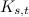$K_{s,t}$.

## Spectrum Results

The spectrum problem for complete bipartite graphs has been completely settled, or almost completely settled, in several small cases, see Table 1. An explanation of the sources of some of these results is given in , with additional results obtained by Emre Kolotoglu . Further existence results are given by Theorem 1, while Theorem 2 provides some non-existence results.

Table 1

 Graph Spectrum Possible Exceptions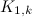$K_{1,k}$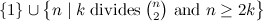$\{1\}\cup\left\{n\mid k\;{\rm divides }\;{n\choose 2}{\rm \ and \ }n\geq 2k\right\}$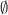$\emptyset$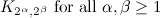$K_{2^{\alpha},2^{\beta}}{\rm \ for \ all \ }\alpha,\beta\geq 1$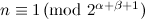$n\equiv 1\,({\rm mod \ }2^{\alpha+\beta+1})$$\emptyset$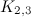$K_{2,3}$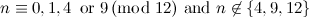$n\equiv 0,1,4\,{\rm \ or \ }9 \,({\rm mod \ }12){\rm \ and \ }n\not\in\{4,9,12\}$$\emptyset$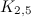$K_{2,5}$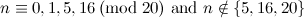$n\equiv 0,1,5,16\,({\rm mod \ }20){\rm \ and \ }n\notin\{5,16,20\}$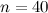$n=40$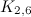$K_{2,6}$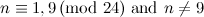$n\equiv 1,9\,({\rm mod \ }24){\rm \ and \ }n\neq 9$$\emptyset$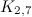$K_{2,7}$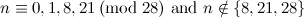$n\equiv 0,1,8,21\,({\rm mod \ }28){\rm \ and \ }n\notin\{8,21,28\}$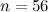$n=56$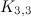$K_{3,3}$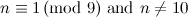$n\equiv 1 \,({\rm mod \ }9){\rm \ and \ }n\neq 10$$\emptyset$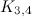$K_{3,4}$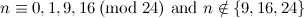$n\equiv 0,1,9,16\,({\rm mod \ }24){\rm \ and \ }n\notin\{9,16,24\}$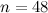$n=48$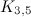$K_{3,5}$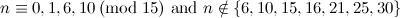$n\equiv 0,1,6,10\,({\rm mod \ }15){\rm \ and \ }n\notin\{6,10,15,16,21,25,30\}$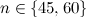$n\in\{45,60\}$

Theorem 1 

There exists a$K_{s,t}$-design of order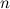$n$ for all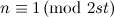$n\equiv 1\,({\rm mod \ }2st)$.

Some non-existence results for$K_{s,t}$-designs have also been proven and are given in Theorem 2.

Theorem 2 , 

Let$s$ and$t$ be any positive integers. There is no$K_{s,t}$-design of order$n$ for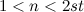$1. Moreover, for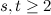$s,t\geq 2$ there does not exist a$K_{s,t}$-design of order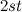$2st$.

## Notes

• In the case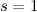$s=1$ we have stars.

## References

1. 1.0 1.1 Adams, P., Bryant, D., and Buchanan, M. A survey on the existence of G-designs, J. Combin. Des. 16, 373–410 (2008).
2. Bermond, J. -C., Huang, C., Rosa, A., and Sotteau, D. Decomposition of complete graphs into isomorphic subgraphs with five vertices, Ars Combin. 10, 211–254 (1980).
3. 3.0 3.1 de Caen, D. and Hoffman, D. G. Impossibility of decomposing the complete graph on n points into n-1 isomorphic complete bipartite graphs, SIAM J. Discrete Math. 2, 48–50 (1989).
4. 4.0 4.1 Graham, R. L. and Pollak, H. O. On embedding graphs in squashed cubes, Graph theory and applications (Proc. Conf., Western Michigan Univ., Kalamazoo, Mich., 1972; dedicated to the memory of J. W. T. Youngs), Springer, Berlin, 99–110. lecture notes in math., vol. 303 (1972).
5. Guy, R. K. and Beineke, L. W. The coarseness of the complete graph, Canad. J. Math. 20, 888–894 (1968).
6. 6.0 6.1 Kolotoglu, E. Decomposition of Complete Graphs into Isomorphic Complete Bipartite Graphs, J. Combin. Des. 21, 524 (2013).
7. 7.0 7.1 Rosa, A. On certain valuations of the vertices of a graph, Theory of Graphs (Internat. Sympos., Rome, 1966), Gordon and Breach, New York, 349–355 (1967).